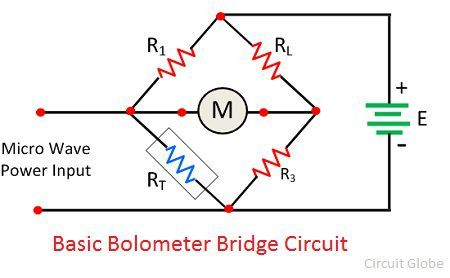# Bolometer

Definition: The bolometer is a device used for detecting and measuring the heat and radiation of  Microwave energy or RF.  It uses the temperature sensitive resistive element who resistance changes with the temperature. The Thermistor and Barretter are the examples of the commonly used resistive element.

The circuit of the bolometer is in the form of the bridge whose one arm contains the temperature sensitive resistor. The temperature sensitive resistor is placed in the field of a microwave energy whose power is to be measured.

The temperature sensitive resistor absorbs the measurand power because of which the heat is generated in it. This heat changes the resistance of an element. The bridge circuit measures the change in resistance. The figure below shows the circuit of the bolometer.The bolometer uses the combination of a bridge circuit as oscillators and a differential amplifier which will oscillate when the bridge is unbalanced. The resistive element of the meter absorbs the power until the bridge becomes balanced.

The DC bias is adjusted for balancing the circuit. The circuit of the bolometer is placed in the field of the microwave signal. The element absorbs the radiation, which increasing their temperature and hence causes the change in their resistance. The variation in resistance makes the bridge unbalanced.The imbalance occurs in the opposite direction because of the cold resistance. The imbalance decreases the output of the oscillator which makes the circuit balance. The electronic voltmeter measures the power decreases from the oscillator. The voltmeter displays the power increases by the oscillator which is absorbed by the resistive element from the microwave field.

The element used by the bolometer bridge is of two types. They are

1. Barretter – It is a metallic wire which has a positive temperature coefficient i.e, their temperature increases with the increases in the temperature.
2. Thermistor – It is a type of thermal resistor made of semiconductor material and has a negative temperature coefficient. The negative temperature coefficient means their resistance decreases with the increases in temperature.

barretters are very delicate as compared to the thermistors. The bolometer is commonly used for measuring the power in the range of 0.01 – 10mW. The combination of bolometer and the attenuators are used for measuring the power above the 10mW.